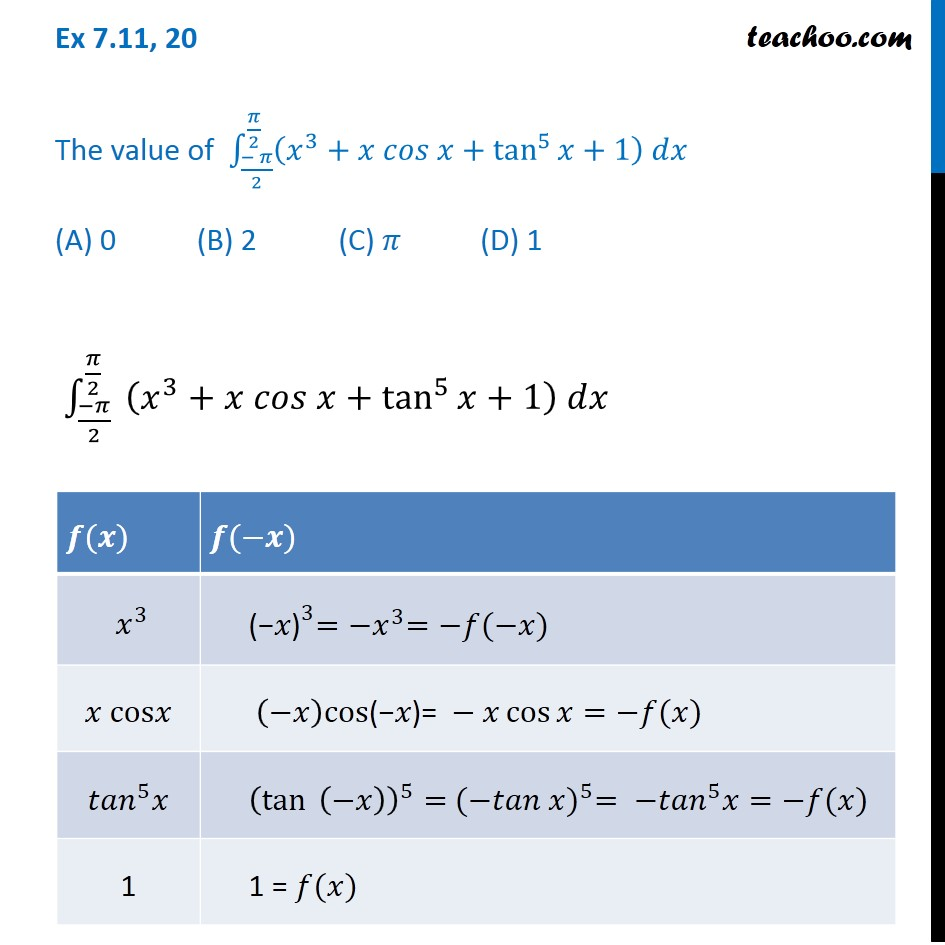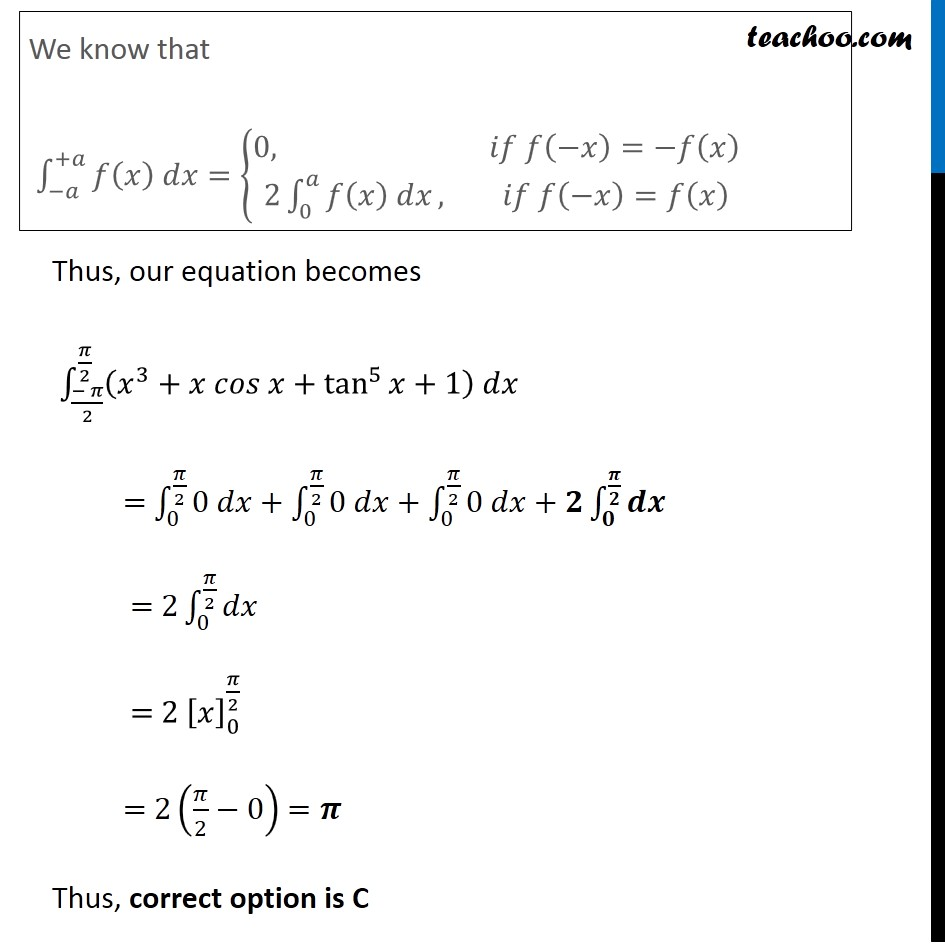Definite Integration by properties - P7

Chapter 7 Class 12 Integrals
Concept wiseIntroducing your new favourite teacher - Teachoo Black, at only ₹83 per month

### Transcript

Ex 7.11, 20 The value of ∫_((− 𝜋)/2)^(𝜋/2)▒〖(𝑥^3+𝑥 𝑐𝑜𝑠 𝑥+tan^5⁡〖𝑥+1〗 ) 𝑑𝑥〗 (A) 0 (B) 2 (C) 𝜋 (D) 1 ∫_((−𝜋)/2)^(𝜋/2)▒〖 (𝑥^3+𝑥 𝑐𝑜𝑠 𝑥+tan^5⁡〖𝑥+1〗 ) 𝑑𝑥〗 We know that ∫_(−𝑎)^(+𝑎)▒〖𝑓(𝑥) 𝑑𝑥〗={█(0, 𝑖𝑓 𝑓(−𝑥)=−𝑓(𝑥)@&2∫_0^𝑎▒〖𝑓(𝑥) 𝑑𝑥〗, 𝑖𝑓 𝑓(−𝑥)=𝑓(𝑥) )┤ Thus, our equation becomes ∫_((− 𝜋)/2)^(𝜋/2)▒〖(𝑥^3+𝑥 𝑐𝑜𝑠 𝑥+tan^5⁡〖𝑥+1〗 ) 𝑑𝑥〗 = ∫_0^(𝜋/2)▒〖0 𝑑𝑥+∫_0^(𝜋/2)▒〖0 𝑑𝑥+∫_0^(𝜋/2)▒〖0 𝑑𝑥+𝟐∫_𝟎^(𝝅/𝟐)▒𝒅𝒙〗〗〗 = 2∫_0^(𝜋/2)▒𝑑𝑥 = 2 [𝑥]_0^(𝜋/2) = 2(𝜋/2−0) =𝝅 Thus, correct option is C